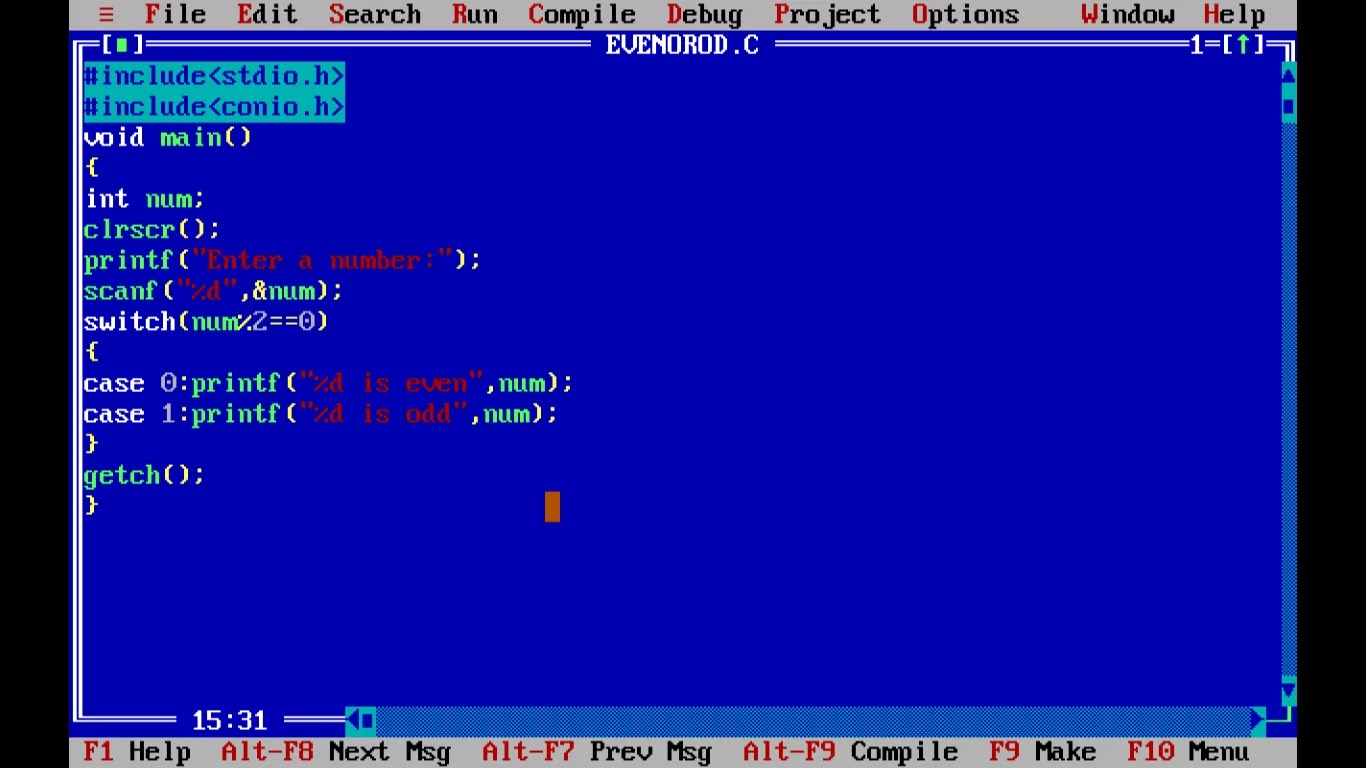# Odd and Even Numbers Program in C

In this program, we are going to make an Odd and Even Numbers Program in C which will check whether the number entered by the user is odd or even. First, we make a C program to check whether the number is odd or even using the if-else statement and in another program, we use a switch-case statement.

## Approach to make Odd and even numbers program in c:

• First, we initialize a number using the int data type.
• We take the value of our num identifier by the user.
• We use the below if-else statement to check whether the number is odd or even

## C program to check whether the number is odd or even using an if-else statement

`#include<stdio.h> #include<conio.h>void main(){int num;clrscr();printf("Enter a number:");scanf("%d",&num);if(num%2==0)printf("%d is even",num);elseprintf("%d is odd",num);getch();}`Output:-
Enter a number:4
4 is even_

### if-else format in the Odd and even numbers program in c program

if(condition) //condition is num%2==0
Statement 1;
else
Statement 2;
If the condition is true then this statement 1 is executed.
if the condition is not true then statement 2 is executed.

## C program to check whether the number is odd or even using a switch-case statement

```#include<stdio.h>
#include<conio.h>
void main()
{
int num;
clrscr();
printf("Enter a number:");
scanf("%d",&num);
switch(num%2==0)
{
case 0:printf("%d is even",num);
break;
case 1:printf("%d is odd",num);
}
getch();
}
```Output:
Enter a number:7
7 is odd_

### FAQ on Odd and even numbers program in c

1. How do you program odd and even numbers?
Even numbers are divisible by 2 means if even numbers are divided by 2 gives a remainder 0. Odd numbers are not divisible by 2 means if odd numbers are divided by 2 gives a remainder 1. When a number is divided by 2, we check the remainder using the modulus operator which is denoted by “%”.

For example num%2 (num can be any natural number)

2. How do you write a c program even or odd?
Odd and even numbers program in c #include<stdio.h>
int main()
{
int a;
printf("Enter any number:");
scanf("%d",&a);
if(a%2==0)
printf("%d is an even number",a);
else
printf("%d is an odd number",a);
return 0;
}

3.How do I make an even odd program in c++?

#include<iostream.h>
int main()
{
int a;
cout<<"\n Enter any number:";
cin>>a;
if(a%2==0)
cout<<a<<" is an even number";
else
cout<<a<<" is an odd number";
return 0;
}

This program is brought to you by Coding Wallah. If you like this program or this code works in your laptop/PC comment below and share your experience. Thanks for reading this blog post. I hope you enjoy this program as much as I enjoy offering them to you. If you have any questions or queries related to this program, please don't hesitate to comment below or contact me. This post is brought to you by coding wallah. Stay safe, stay connected with us.• Hackerrank 5* • Full Stack Developer • Masters in SEO

### 1 comment

1.Can we make odd even number program in c using another method?